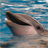# OD Cost Matrix for symmetric distances

177
2
05-01-2012 09:03 AMNew Contributor II
Hello all,

For research purposes, I am currently solving OD Cost Matrix to compute shortest paths distances for about 10'000 origins and destinations (origins = destinations). I am using a undirected network, so the distance from A to B equal distance from B to A (symmetric distances). Therefore, do anyone has an idea on how to customize the OD Cost Matrix tool so that it only computes A to B and not B to A ? This would halve the size of the database and the computing time.

Any idea ?
Best regards,
Theo
Tags (2)
2 RepliesNew Contributor II

Theo

Hello all,

For research purposes, I am currently solving OD Cost Matrix to compute shortest paths distances for about 10'000 origins and destinations (origins = destinations). I am using a undirected network, so the distance from A to B equal distance from B to A (symmetric distances). Therefore, do anyone has an idea on how to customize the OD Cost Matrix tool so that it only computes A to B and not B to A ? This would halve the size of the database and the computing time.

Any idea ?
Best regards,
Theoby
Occasional Contributor II
Hallo,

I am assuming that your O and D are same in number and same in locations. I am also assuming that OD cost matrix can be called with a python script. If above two conditions are true, then a small code "if j > i then calculate distance" will give the required result, where Ai is set of O and Bj is set of D and i is exact same quantity as j, because number of O = number of D. This will give you matrix values above the diagonal, that is one half of the matrix (diagonal being zero of course)

Regards

Hello all,

For research purposes, I am currently solving OD Cost Matrix to compute shortest paths distances for about 10'000 origins and destinations (origins = destinations). I am using a undirected network, so the distance from A to B equal distance from B to A (symmetric distances). Therefore, do anyone has an idea on how to customize the OD Cost Matrix tool so that it only computes A to B and not B to A ? This would halve the size of the database and the computing time.

Any idea ?
Best regards,
Theo